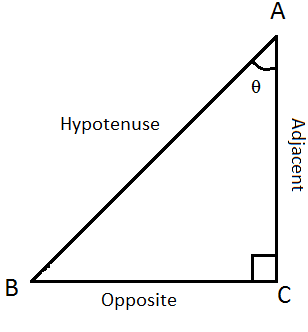## Meaning of Trigonometry

Many people give up on learning trigonometry right after hearing the name...
"Trigono" is Greek for triangle and "metron" means measure. So basically trigonometry deals with the relationship between lengths and angles of a triangle. So now that we've got the meaning out of the way, lets start with the 3 basic functions of trigonometry.

## The 3 Basic Trigonometry functions

In this article we'll only deal with trigonometry with acute angles and right triangles.
There are 3 basic trigonometry ratios :-
• Sine (sin)
• Cosine (cos)
• Tangent (tan)

Before actually learning what these functions mean, we should learn some terminology.

For any acute angle θ in a right triangle:
• The side opposite to θ is called 'Opposite' side
• The longest side, that is the side opposite the right angle is called the 'hypotenuse'
*Note- Remember that the Adjacent and the Opposite sides change relativeNow that we know what all sides relative to an angle in a right triangle are called, lets define the 3 trigonometric functions.

The sine of an acute angle in a right triangle is defined as the length of the side opposite said angle over the hypotenuse.

Sin θ =   Opposite
Hypotenuse

The cosine of an acute angle in a right triangle is defined as the length of the adjacent side over the hypotenuse.

Hypotenuse

The Tangent of an acute angle in a right triangle is defined as the length of the opposite side over the adjacent side

Tan θ =   Opposite

Do you think you wont be able to remember these 3 ratios?
well just remember the mnemonic SohCahToa
Soh - Sine is Opposite/Hypotenuse

Soh,(no pun intended) now if someone asks you what the sine of 60゜ is by looking at the triangle below, this is how you would do it
you just say that as sine of an angle is Opposite/Hypotenuse and as the length of the side opposite to the 60゜ angle is √3 units and the length of the hypotenuse is 2 units.
The sine of 60゜= √3/2 and this will be true in all triangles, sin 60 will always be equal to √3/2 . This means the ratio of the opposite side to the 60゜ angle to the hypotenuse in a right triangle will always be √3/2. Lets see why...

## Why are trigonometric function of an angle constant!?

To prove this we can show that sine, cosine or tangent of θ in triangles with different length of sides will be equal.
sin θ(ABC) = b/c
sin θ (DEF) = e/f
As we know that the sum of the angles in a triangle = 180゜
So, θ + 90゜ + A = 180゜
subtract 90゜ from both sides
θ + A = 90゜
A = 90゜- θ
So, now that we know that all 3 angles in ABC are equal to corresponding angles in DEF
∴ △ABC ∼ △DEF
as the triangles are similar the ratio of their corresponding sides is equal
That means, a/d = b/e = c/f
if we substitute 'k' for the constant of ratio
then, d . k = a
f . k = c
e . k = b

sin θ = b/c = e . k/ f . k
sin θ = b/c = e/f
Similarly we can prove this for the cosine and tangent also.

This is how far we will go into trigonometry in this article, if you have any doubts or questions related to trigonometry I'll answer them in the comments section below, in the next post, We will deal with trigonometry with general triangles and the reciprocal Trigonometric functions, Until then, Stay TUNED !   :D

### You Might Also Like :-

Enjoy your high school with - High School Pedia : www.highschoolpedia.com

### Cathode Ray Experiment

This experiment was conducted by J.J. Thomson (Sir Joseph John Thomson) in the year 1897. This experiment proved that atom is made up of fundamental particles which are much smaller than the smallest atom 'hydrogen' This experiment helped to discover electron. According to J.J. Thomson, the cathode rays consisted of very light, small and negatively charged particles. He named the particles "corpuscles" which were later known as electrons

### Isotopes, Isobars and Isotones

Isotopes These are elements which have the same atomic number but different atomic mass . They have the same atomic number because the number of protons that are inside their nuclei remains the same. But, they have different atomic mass because the number of neutrons that are also inside their nuclei is different. As the number of protons inside nuclei remains same, therefore the overall charge of the elements also remains same as in isotopes: no of protons = no of electrons . Hence, as isotopes overall charge remains neutral, therefore their chemical properties will also remain identical.   Therefore, Isotopes are chemically same but physically different.